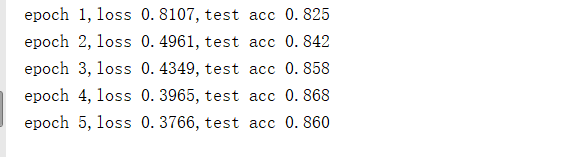```from mxnet import gluon,init
from mxnet.gluon import loss as gloss, nn
from mxnet.gluon import data as gdata
import gluonbook as gb

import sys

# 讀取資料
# 讀取資料
mnist_train = gdata.vision.FashionMNIST(train=True)
mnist_test = gdata.vision.FashionMNIST(train=False)

batch_size = 256
transformer = gdata.vision.transforms.ToTensor()
if sys.platform.startswith('win'):
num_workers = 0
else:
num_workers = 4

# 小批量資料迭代器

# 定義網路
net = nn.Sequential()
net.initialize(init.Normal(sigma=0.01))

# 損失函式
loss = gloss.SoftmaxCrossEntropyLoss()
trainer = gluon.Trainer(net.collect_params(),'sgd',{'learning_rate':0.5})

def accuracy(y_hat, y):
return (y_hat.argmax(axis=1) == y.astype('float32')).mean().asscalar()

def evaluate_accuracy(data_iter, net):
acc = 0
for X, y in data_iter:
acc += accuracy(net(X), y)
return acc / len(data_iter)

num_epochs = 5

def train(net,train_iter,test_iter,loss,num_epochs,batch_size,params=None,lr=None,trainer=None):
for epoch in range(num_epochs):
train_l_sum = 0
train_acc_sum = 0
for X,y in train_iter:
y_hat = net(X)
l = loss(y_hat,y)
l.backward()

if trainer is None:
gb.sgd(params,lr,batch_size)
else:
trainer.step(batch_size)

train_l_sum += l.mean().asscalar()

test_acc = evaluate_accuracy(test_iter,net)
print('epoch %d,loss %.4f,test acc %.3f'%(epoch+1,train_l_sum / len(train_iter),test_acc))

train(net,train_iter,test_iter,loss,num_epochs,batch_size,None,None,trainer)```